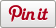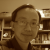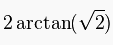# Methane Molecule

•• Sharebar
•This is my first and simple construction of a molecule. I chose to do Methane (CH4), one atom of carbon and four atoms of hydrogen, because it takes the shape of a tetrahedron. In this blog, I used a different but simple method to construct this tetrahedron shape. There is a special angle in tetrahedron. With this special angle, we can easily construct a tetrahedron.

This special angle is about 109.4712 degrees. It is the Edge central angle, also known as the tetrahedral angle or bond angle of the methane molecule. To be precise, this angle can be calculated asor.

Below is the 3D model created in VRMath2 Editor. I added a basic animation so the molecule will be rotating. You can click on the Carbon atom at the centre to toggle on and off the animation.

The Logo program is as below.